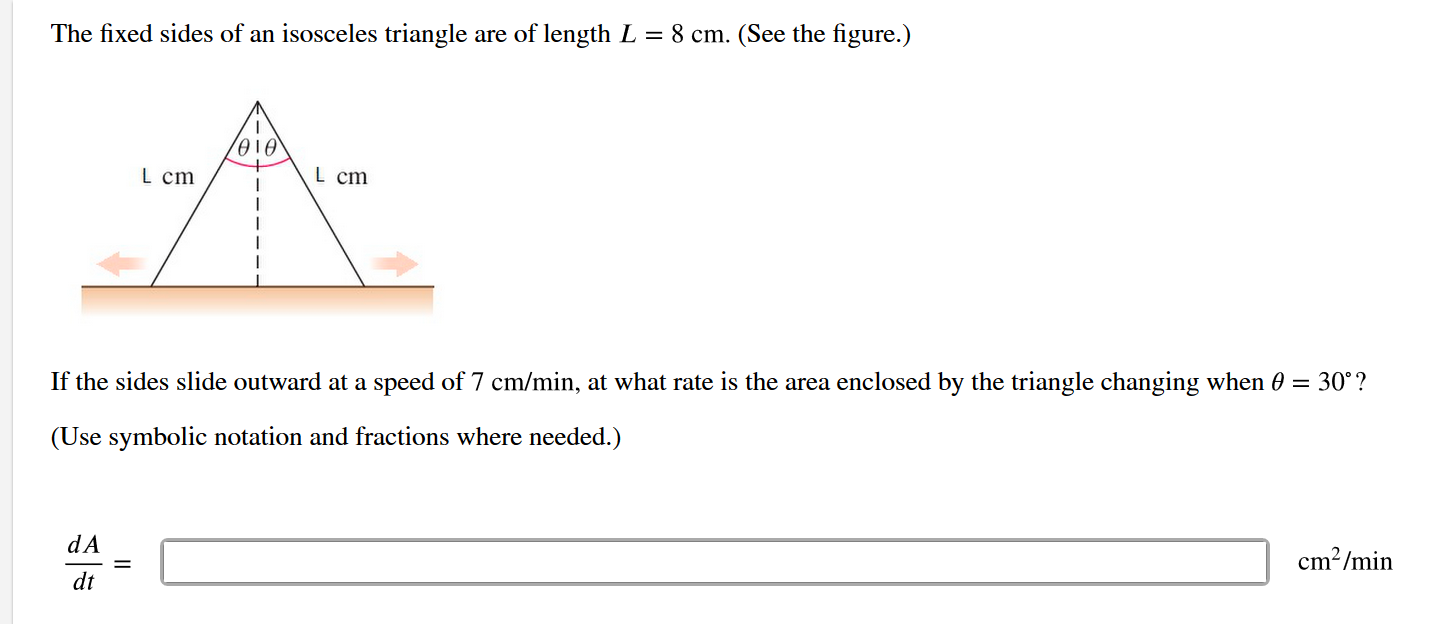# The fixed sides of an isosceles triangle are of length L = 8 cm. (See the figure.)L cmL cmIf the sides slide outward at a speed of 7 cm/min, at what rate is the area enclosed by the triangle changing when 0 = 30°?(Use symbolic notation and fractions where needed.)cm2/mindAdtII

Question
34 viewshelp_outlineImage TranscriptioncloseThe fixed sides of an isosceles triangle are of length L = 8 cm. (See the figure.) L cm L cm If the sides slide outward at a speed of 7 cm/min, at what rate is the area enclosed by the triangle changing when 0 = 30°? (Use symbolic notation and fractions where needed.) cm2/min dA dt II fullscreen
check_circle

Step 1

Given, length of isosceles triangle is 8 cm. Two sides are equal which is AB and AC.

Step 2

Sides sliding outward at a speed of

Step 3

Area of triangle i...

### Want to see the full answer?

See Solution

#### Want to see this answer and more?

Solutions are written by subject experts who are available 24/7. Questions are typically answered within 1 hour.*

See Solution
*Response times may vary by subject and question.
Tagged in

### Derivative